# Membrane X and triangular elements

Last reviewed version: 2.17.2

## Introduction

With the introduction of AquaSim 2.17.2 the handling of membranes was further improved upon. This has meant that tricks to create triangular elements with 4 noded membrane elements (which up until recently was the only way to model membranes) are no longer valid.

The help this situation we have introduced the Membrane X (for extended) component group. It shares all properties with the regular Membrane component except for how it is generated and modelled. The Membrane component consists of lines which are then turned into 4 noded elements by an algorithm. Membrane X, on the other hand, lets the user draw the individual membranes themselves.

This allows Membrane X to allow 3 noded elements, and to create geometries where the original algorithm would fail to generate a good set of geometry (see areas of issues further down).

## Indication

During check model the error message “Membrane element too triangular, consider using triangular membrane x triangle element” appears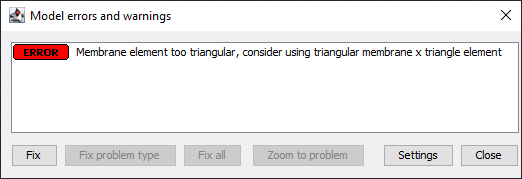This error message is cause by two adjoining lines in the element being (close to) parallel. In this example element A and B are parallel, causing the problematic geometry.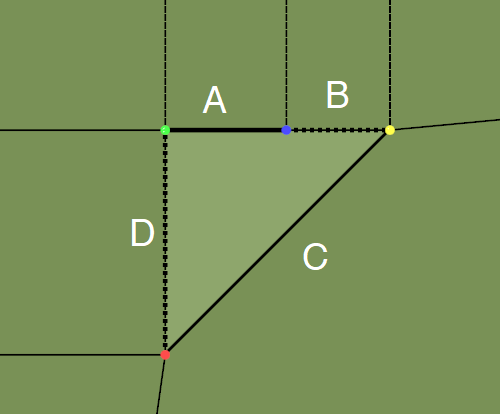Also note that twine directions will not be accurate when using highly deformed quads (4 noded elements). See note at the end of this document.

## Solution

The correct solution to this problem is to convert the component to membrane x (note: Membrane components can be converted to membrane x, keeping all generated geometry), and using the “Draw membrane” tool which has an option to enable Triangle mode.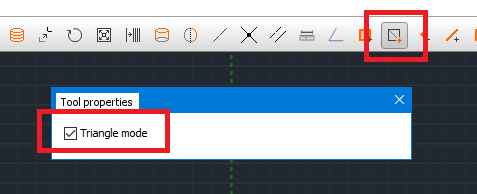Removing the offending element first and redrawing it as two triangles produces this (valid) result: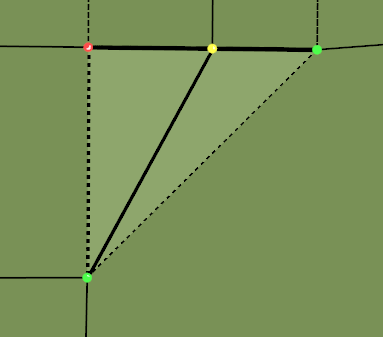## Note on twine direction

In quad elements, the twines are organized in the same manner as Mask Type 1 for conventional membranes. The twines will cross perpendicular to the horizontal edge of the quad element. This is illustrated to the left in the figure below, where the green grid representing the twines, and the blue lines are the quad element. The dashed line is the horizontal edge of the quad element, and the solid line is the vertical edge.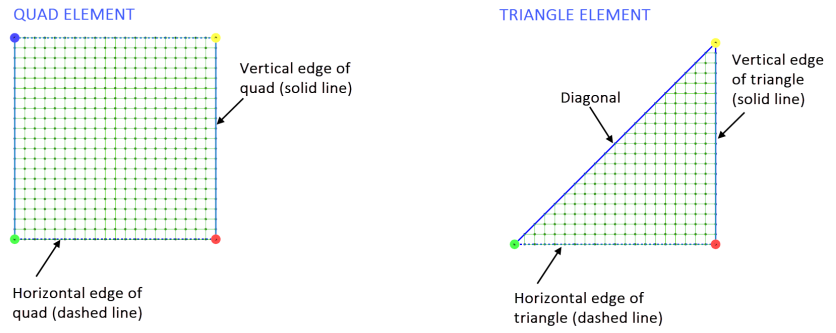Triangle elements are similar ), the twines cross perpendicular to the horizontal edge of the triangle element. The diagonal (i.e., solid line between green and yellow node) will cross the twine’s diagonal.